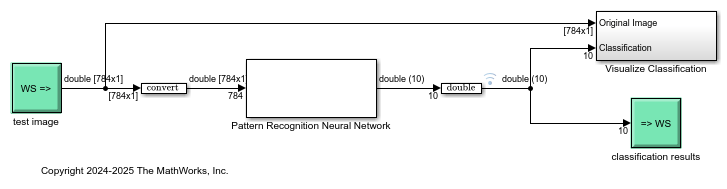# Digit Classification with Half-Precision Data Types

This example compares the results of a trained neural network classification model in Simulink® in double precision and half precision. The model classifies images from the MNIST handwritten digit dataset.

To begin, load the data for the model, and specify the size of the test data set.

```load trainImage.mat testSetMaxIdx = 10; ```

### Simulate the Model with Double-Precision Types

This model uses `numerictype` objects to specify parameter, signal, and block output data types. To simulate the model using double-precision data types, define the `numerictype` objects in the base workspace and set the data type of the objects to `'double'`. Simulate the model.

```floatType = numerictype('double'); activationType = numerictype('double'); model = 'ex_imagerecog_half.slx'; open_system(model); sim(model); ```The double-precision simulation results in 100% classification accuracy.

```[c, ~] = confusion(ttestsubset(:,1:testSetMaxIdx), ytest.Data(2:testSetMaxIdx+1,:)'); fprintf('Percentage Correct Classification : %f%%\n', 100*(1-c)); fprintf('Percentage Incorrect Classification : %f%%\n', 100*c); ```
```Percentage Correct Classification : 100.000000% Percentage Incorrect Classification : 0.000000% ```

### Simulate the Model with Half-Precision Types

To simulate the model in half precision, redefine the `numerictype` objects and set their data type to `'half'`. Simulate the model.

```floatType = numerictype('half'); activationType = numerictype('single'); sim(model); ```

In this example, there is no loss of accuracy when using the half-precision data type. The half-precision simulation also results in 100% classification accuracy.

```[c, ~] = confusion(ttestsubset(:,1:testSetMaxIdx), ytest.Data(2:testSetMaxIdx+1,:)'); fprintf('Running Simulation with half precision :\n'); fprintf('Percentage Correct Classification : %f%%\n', 100*(1-c)); fprintf('Percentage Incorrect Classification : %f%%\n', 100*c); ```
```Running Simulation with half precision : Percentage Correct Classification : 100.000000% Percentage Incorrect Classification : 0.000000% ```

## SupportGet trial now